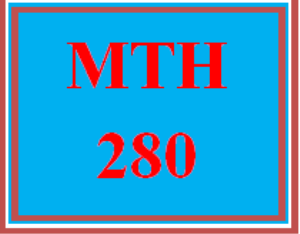# MTH 280 Wk 4 – Midterm Exam

PLDZ-13038 Free In Stock
\$ 0.00 USD
Description

***************************************************************

https://uopcourse.com/category/mth-280/ ~~~~~~~~~~~~~~~~~~~~~~~~~~~~~~~~~~~~~~~~~~~~~~~~~

https://uopcourse.com/

***************************************************************

# MTH 280 Wk 4 – Midterm Exam

Question 1

A research lab grows a type of bacterium in culture in a circular region. The radius of the circle, measured in centimeters, is given by begin mathsize 12px style r left parenthesis t right parenthesis space equals space 5 space minus space fraction numerator 6 over denominator t squared plus 3 end fraction end style, where t is time measured in hours passed since a circle of a 1cm radius of the bacterium was put into the culture. Express the area, A open parentheses t close parentheses, of the bacteria as a function of time, and find the approximate area of the bacterial culture in 4 hours.

A open parentheses t close parentheses space equals space pi left parenthesis 5 minus fraction numerator 6 over denominator t squared plus 3 end fraction right parenthesis and Area after 4 hours = 14.72 comma space c m squared

A open parentheses t close parentheses space equals space 2 pi left parenthesis 5 minus fraction numerator 6 over denominator t squared plus 3 end fraction right parenthesis and Area after 4 hours = 29.43 comma space c m squared

A open parentheses t close parentheses space equals space pi left parenthesis 5 minus fraction numerator 6 over denominator t squared plus 3 end fraction right parenthesis and Area after 4 hours = 68.93 comma space c m squared

A open parentheses t close parentheses space equals space 2 pi left parenthesis 5 minus fraction numerator 6 over denominator t squared plus 3 end fraction right parenthesis and Area after 4 hours = 137.86 comma space c m squared

Question 2

A minivan was purchased for \$32,000. If the value of the minivan depreciates by \$1,700 per year, find a linear function that models the value V of the car after t years. Use the function and find the value of the car after 5 years.

V(t) = -1,700t + 32,000; value after 5 years = \$23,500

V(t) = -1,700 + 32,000; value after 5 years = \$30,300

V(t) = 1,700 + 32,000; value after 5 years = \$33,700

V(t) = 1,700t + 32,000; value after 5 years = \$40,500

Question 3

Simplify tan x left parenthesis c s c x space minus space sin x right parenthesis space.

1

sin x

cos x

Question 4

A pendulum moving in simple harmonic motion is modelled by the function s open parentheses t close parentheses equals negative 5 cos left parenthesis begin inline style fraction numerator pi t over denominator 4 end fraction end style right parenthesis , where s is measured in inches and t is measured in seconds. Determine the first time when the distance moved is 4 inches.

Recent Reviews Write a Review
0 0 0 0 reviews